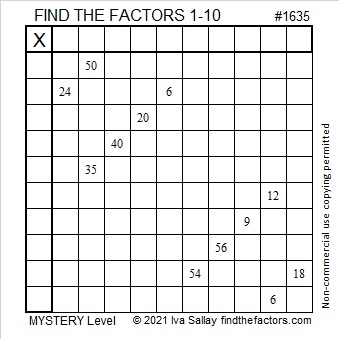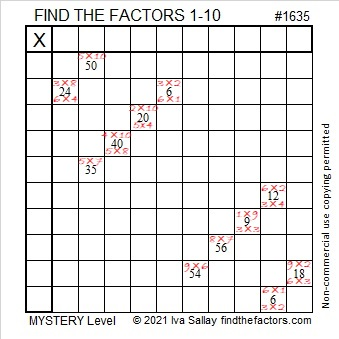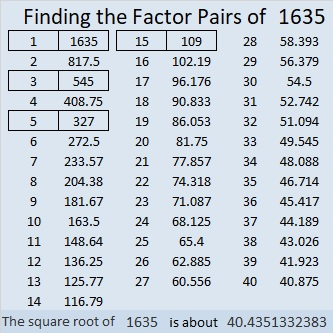# 1635 The Logic to This Puzzle Is a Real Mystery

### Today’s Puzzle:

You can easily find a way to start this puzzle, but just a few factors later, it’s a mystery what to do next. Give it a shot, and see what I mean.Writing all the factor pairs for the clues often is helpful,but not as helpful as we might hope this time. Here’s a video explaining what to do to find a few more factors of this puzzle using logic:

### Factors of 1635:

• 1635 is a composite number.
• Prime factorization: 1635 = 3 × 5 × 109.
• 1635 has no exponents greater than 1 in its prime factorization, so √1635 cannot be simplified.
• The exponents in the prime factorization are 1, 1, and 1. Adding one to each exponent and multiplying we get (1 + 1)(1 + 1)(1 + 1) = 2 × 2 × 2 = 8. Therefore 1635 has exactly 8 factors.
• The factors of 1635 are outlined with their factor pair partners in the graphic below.### More about the Number 1635:

1635 is the hypotenuse of FOUR Pythagorean triples:
99-1632-1635, which is 3 times (33-544-545),
552-1539-1635, which is 3 times (184-513-545),
900-1365-1635, which is 15 times (60-91-109), and
981-1308-1635, which is (3-4-5) times 327.

OEIS.org informs us that there’s something special about the first nine decimals places of the fifth root of 1635.
Its fifth root is 4.392416875…
Can you figure out what is so special about that?